# 關係&函數（Relations & Functions）

## 二元關係（binary relation）

### 二、多個集合的二元關係

#### 「多個集合二元關係」範例

「ρ」必為「S×T={(0,-1),(0,-2),(0,-3),(1,-1),(1,-2),(1,-3),(2,-1),(2,-2),(2,-3),(3,-1),(3,-2),(3,-3)}」的子集，而在 S×T 中，有哪些元素符合「x+y=0」條件呢？

### 三、齊次關係（homogeneous relation）

#### 自反性（reflexive）

[∀(x)∈ S]‧[(x,x) ∈ ρ]

#### 非自反性（irreflexive）

[∀(x)∈ S]‧[(x,x) ∉ ρ]

#### 對稱性（symmetric）

[∀(x,y)∈ S]‧{[(x,y)∈ ρ] → [(y,x)∈ ρ]}

#### 反對稱性（antisymmetric）

[∀(x,y)∈ S]‧{[(x,y)∈ ρ ∧ (y,x) ∈ ρ] → (x=y)}

#### 非對稱性（asymmetric）

[∀(x,y)∈ S]‧[(x,y)∈ ρ → (y,x) ∉ ρ]

#### 遞移性（transitive）

[∀(x,y,z)∈ S]‧[(x,y)∈ ρ ∧ (y,z) ∈ ρ→ (x,z) ∈ ρ]

#### 弱連通性（connected）

[∀(x,y)∈ S]‧{(x≠y) → [(x,y)∈ ρ] ∨ [(y,x)∈ ρ]}

#### 連通性／全關係／完全性（strongly connected / totality）

[∀(x,y)∈ S]‧[(x,y)∈ ρ) ∨ (y,x)∈ ρ)]

## 五、偏序關係（partial order relation）

1. 自反性（reflexive）
2. 反對稱性（antisymmetric）
3. 遞移性（transitive）

1. 自反性：在 R 中，任意數必「≤」自己，因此具有自反性。
2. 反對稱性：在 R 中，如果 x≤y 又 y≤x，則 x=y，因此具有反對稱性。
3. 遞移性：在 R 中，如果 x≤y 又 y≤z，則 x≤z，因此具有遞移性。

「x≤y」在 R 中同時具備自反性、反對稱性與遞移性，因此可說「≤」這個二元關係為一「偏序關係」。

#### 哈斯圖（Hasse diagram）

1. 由下而上標示集合中各個元素。
2. 繪製各相同與相異元素間「有向圖（directed graph）」。
3. 移除所有表示「自反性」的箭頭小圈。
4. 移除所有表示「遞移性」的長箭頭。
5. 因所有箭頭方向皆為由下往上，可將所有箭頭改為無方向性的線段。

1. 由下而上依序標示元素 1、2、3、4。
2. ρ 包含 {(1,1),(1,2),(1,3),(1,4),(2,2),(2,4),(3,3),(4,4)}，將這些二元關係全部以箭頭表示。
3. 移除所有表示「自反性」關係的小圈箭頭，包含 {(1,1),(2,2),(3,3),(4,4)}，ρ 集合中剩下 {(1,2),(1,3),(1,4),(2,4)}。
4. 移除所有表示「遞移性」的長箭頭，因為 1 可以連到 2、2 也能連到 4，因此移除 {(1,4)}，此時 ρ 集合中剩下 {(1,2),(1,3),(2,4)}。
5. 因所有箭頭方向皆往上，故可移除所有箭頭。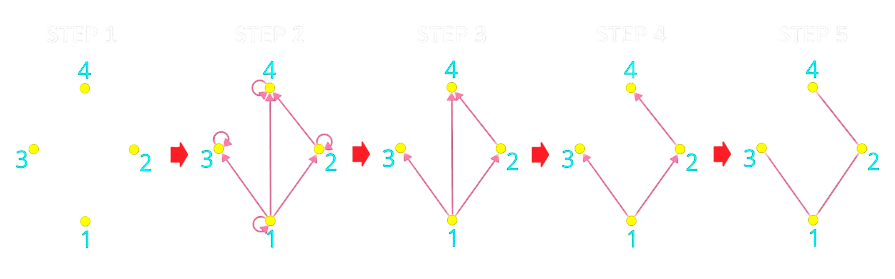#### 實質等價／若且唯若（equivalence / if and only if）

1. 自反性（reflexive），同「偏序關係」
2. 對稱性（symmetric），「偏序關係」為「反對稱性」
3. 遞移性（transitive），同「偏序關係」

1. 自反性：在 R 中，任意數必「=」自己，因此具有自反性。
2. 對稱性：在 R 中，如果 x=y，則 y=x，因此具對稱性。
3. 遞移性：在 R 中，如果 x=y 又 y=z，則 x=z，因此具有遞移性。

「x=y」在 R 中同時具備自反性、對稱性與遞移性，因此可說「=」這個二元關係為一「實質等價關係」。

## 函數（function）

1. 自變數（independent variable）：函數輸入值，如 f(x) 函數的 x。
2. 應變數（dependent variable）：函數輸出值，如 x 經 f(x) 函數得到的值。
3. 原像（preimage）：經函數逆向處理的結果，相當於自變數。
4. 像（image）：經函數順向處理的結果，相當於應變數。
5. 定義域（domain）：自變數的集合。
6. 對應域（codomain）：應變數存在的集合，為值域的超集，當中不屬於值域的元素無法在定義域中找到可對應的自變數。
7. 值域（range）：應變數的集合，為對應域的子集。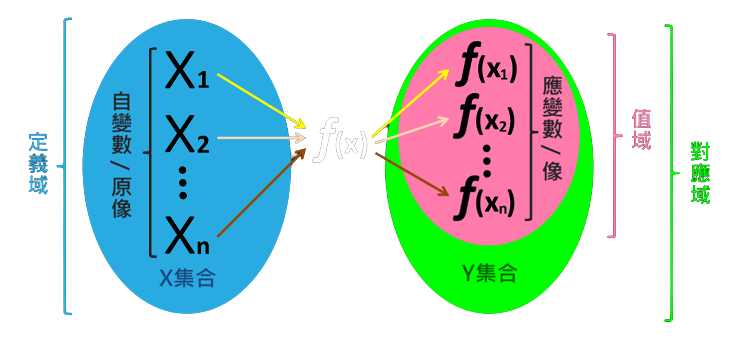f(x) 函數將 X 集合中的「原像」對應到 Y 集合中的「像」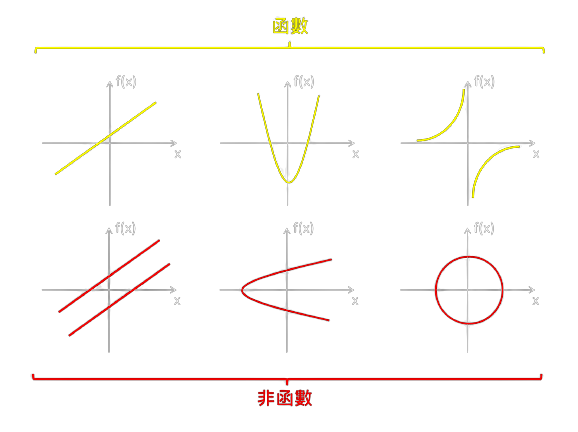### 一、單射／嵌射（one-to-one / injection）

∀(a,b)∈ X，f(a)=f(b) → a=b

∀(a,b)∈ X，a≠b → f(a)≠f(b)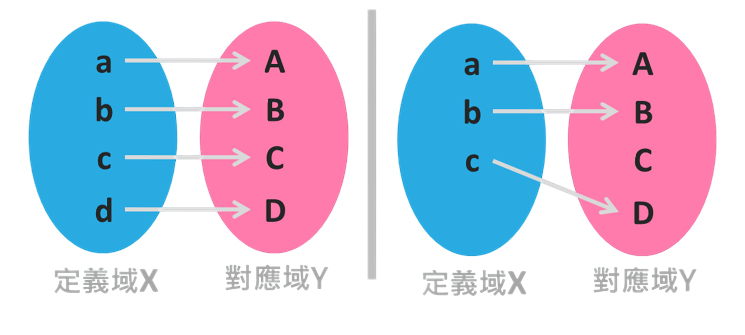#### 單射函數個數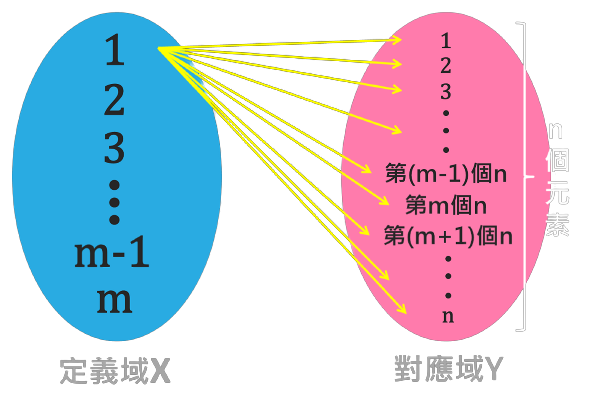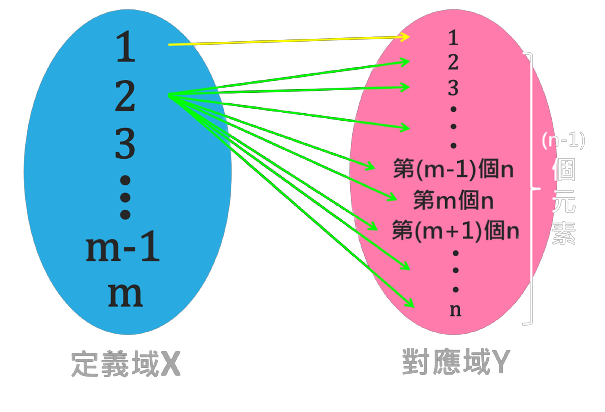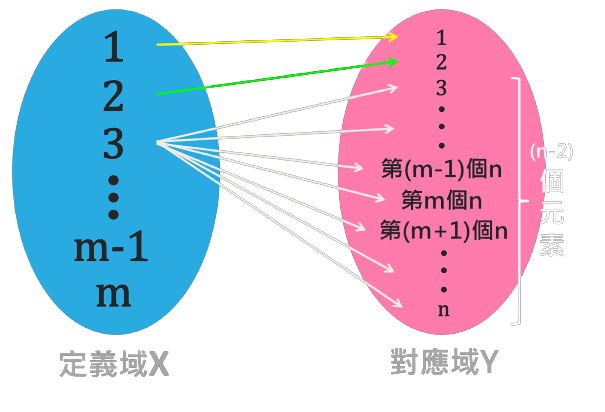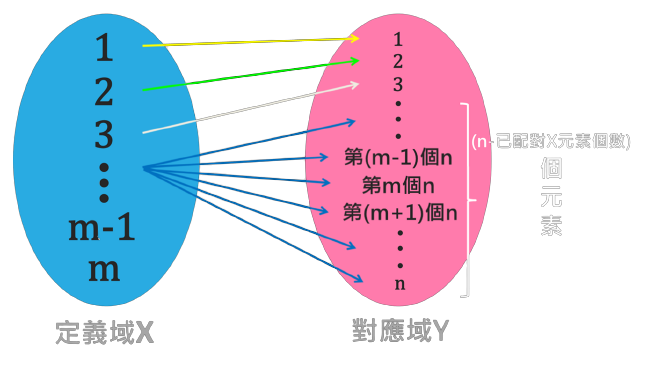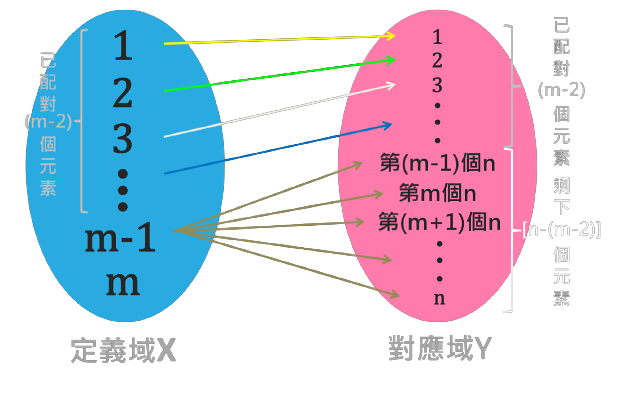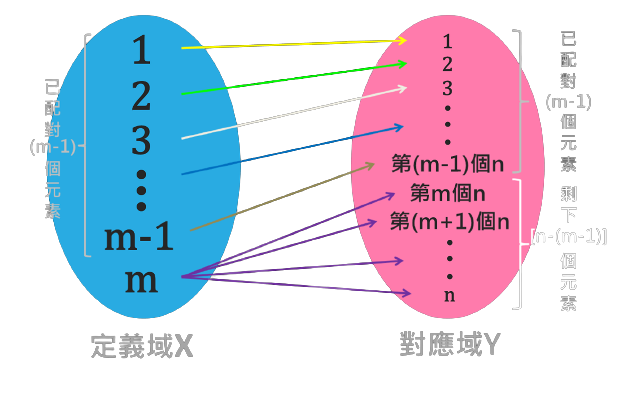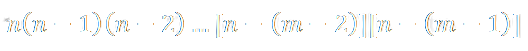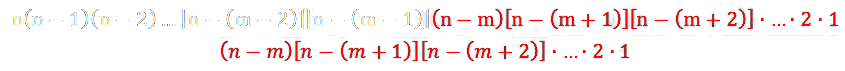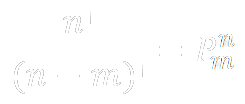### 二、滿射／蓋射（onto / surjection）

(∀y∈ Y), (∃x∈ X), f(x)=y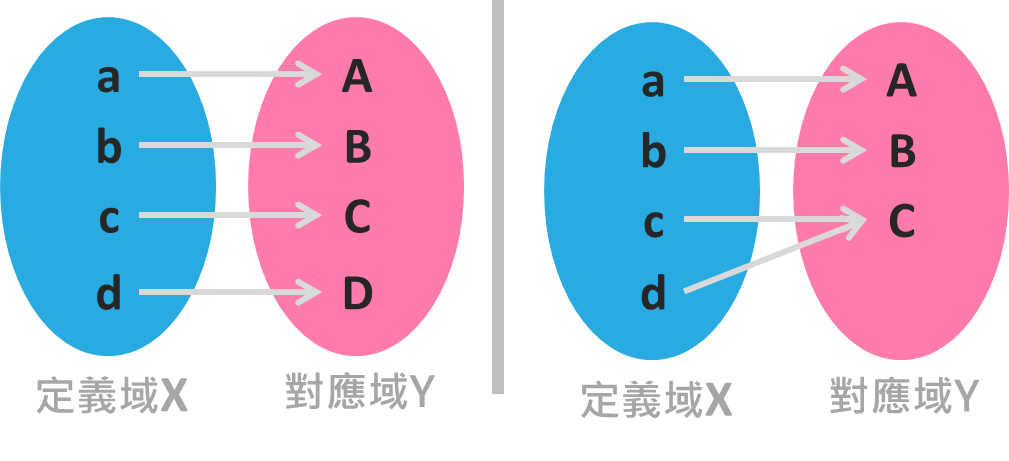#### 滿射函數個數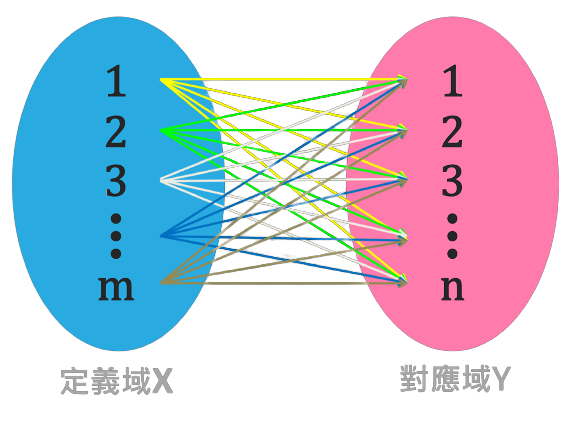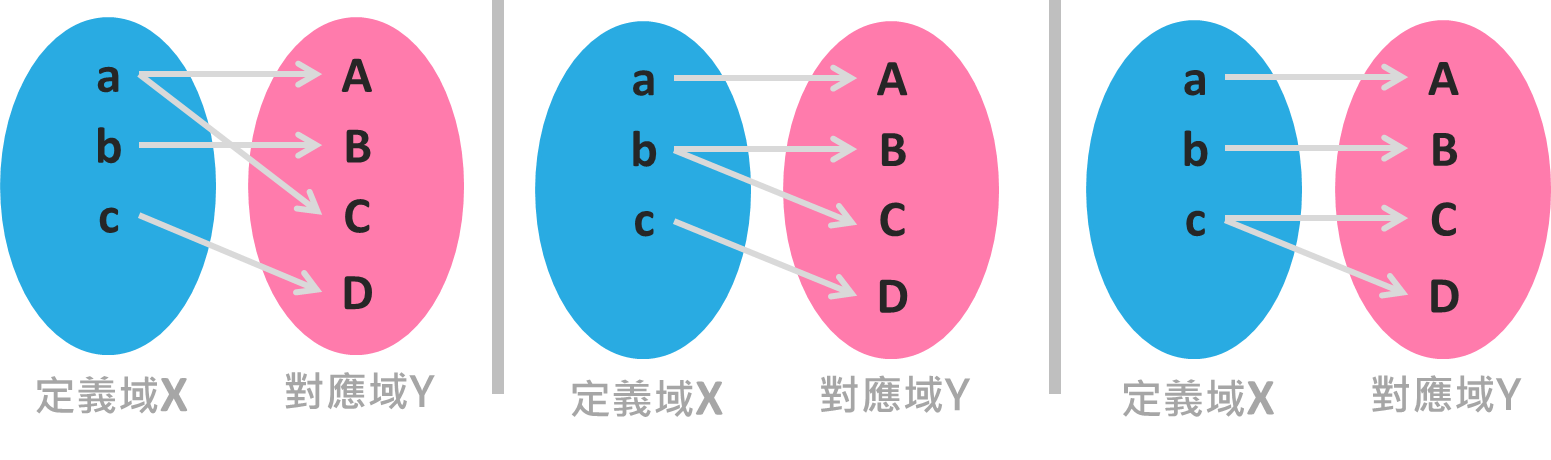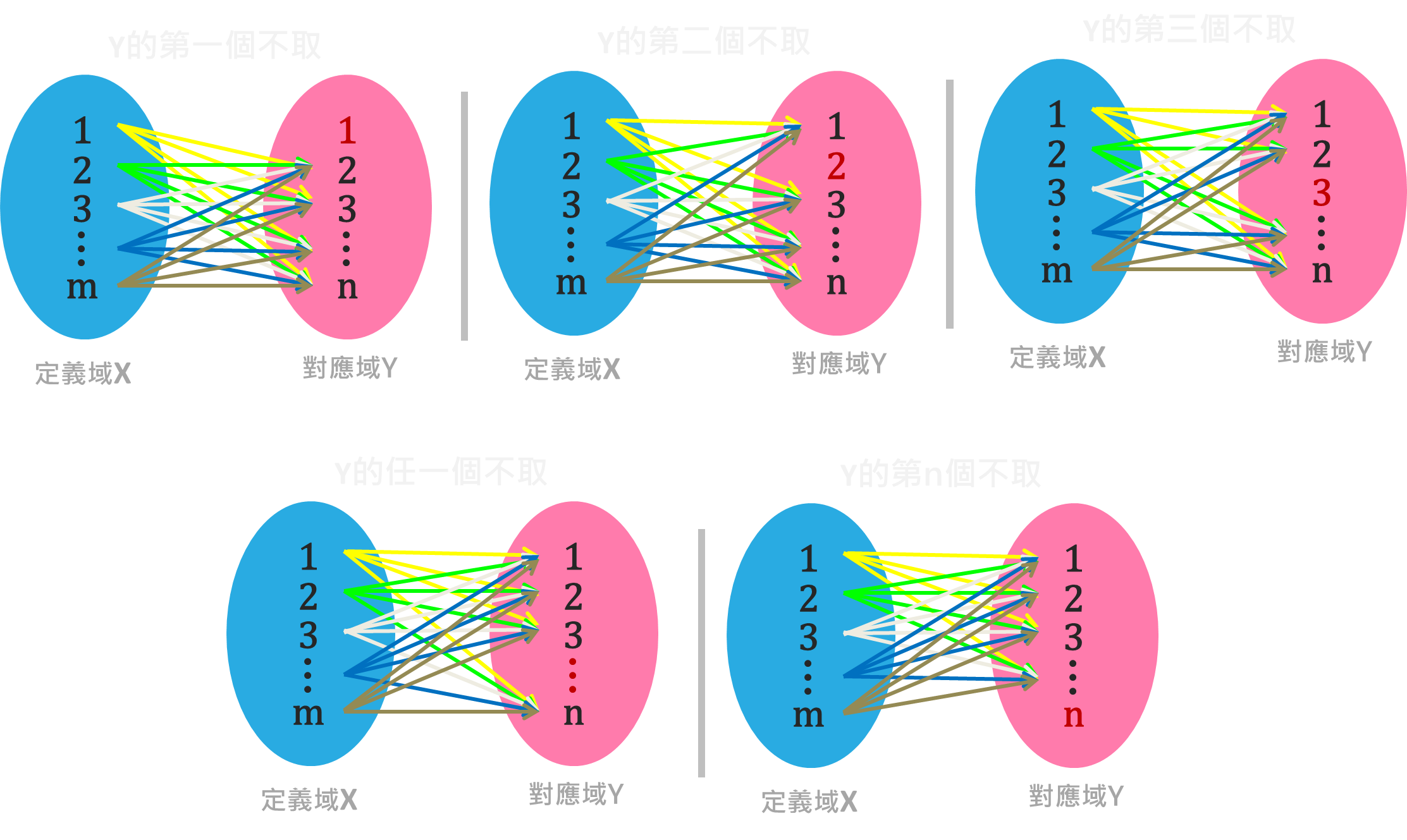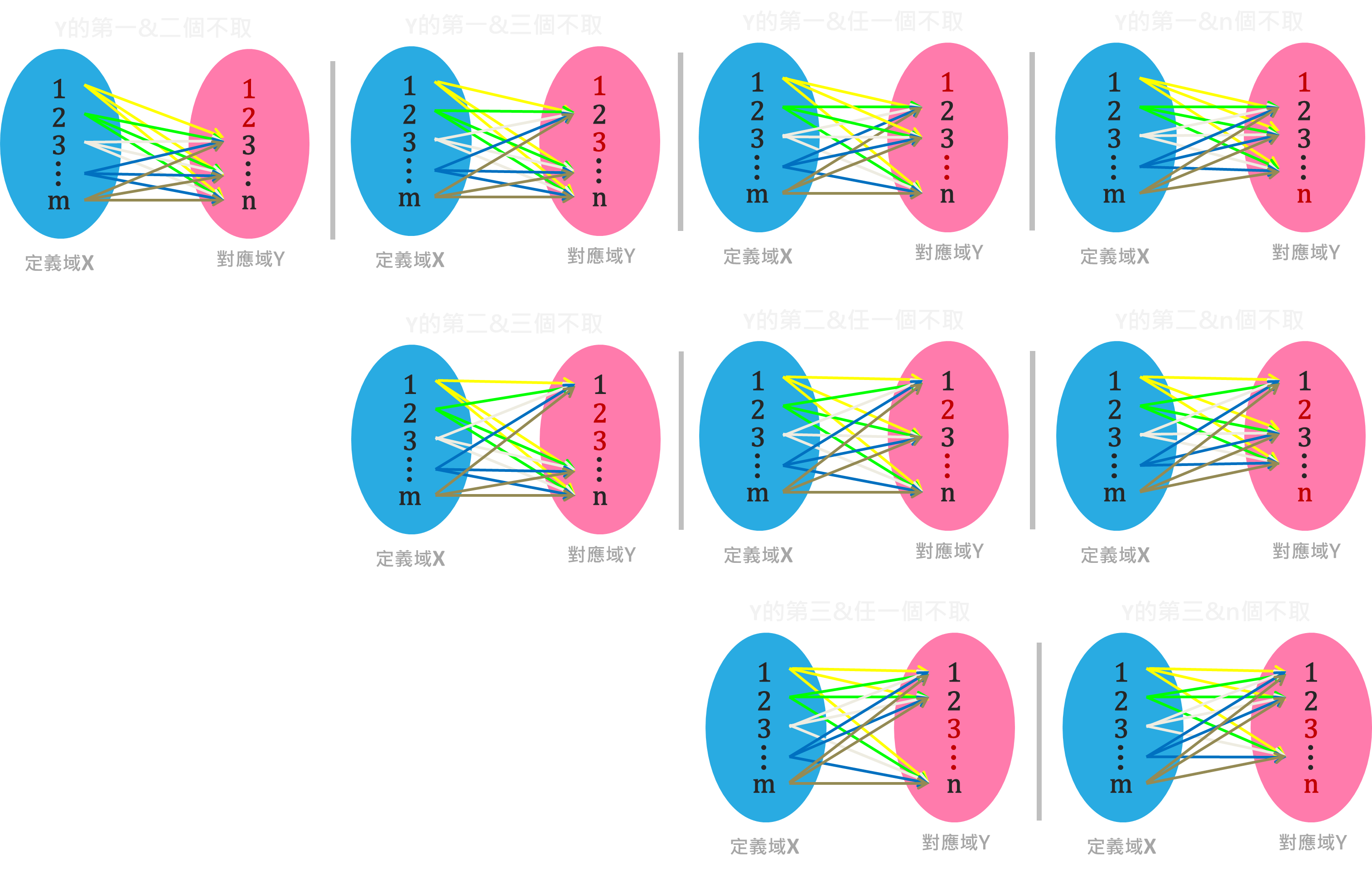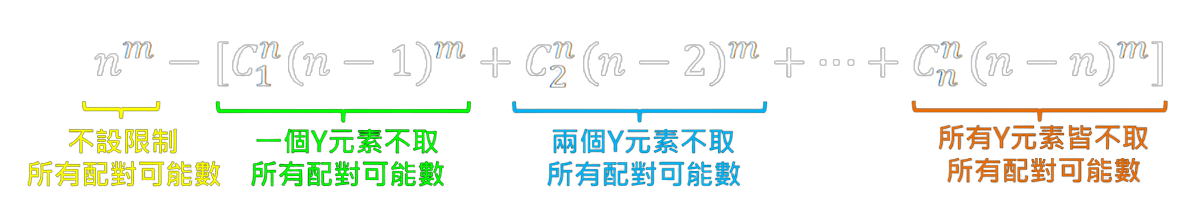### 三、雙射／對射（bijection）

1. 每個 X 中的元素必至少與一個 Y 中的元素配對。
2. 每個 X 中的元素必不與超過一個 Y 中的元素配對（即「單射」）。
3. 每個 Y 中的元素必至少與一個 X 中的元素配對（即「滿射」）。
4. 每個 Y 中的元素必不與超過一個 X 中的元素配對。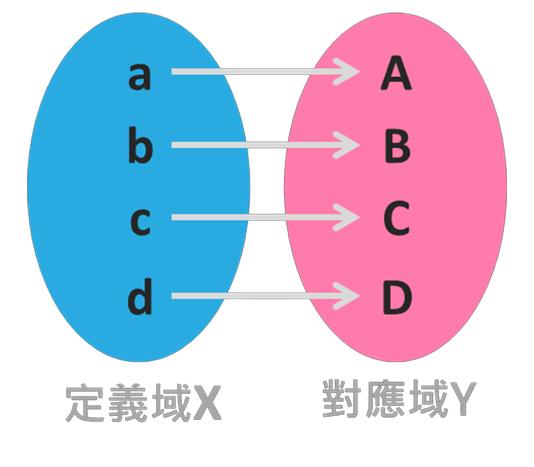### 四、反函數（inverse function）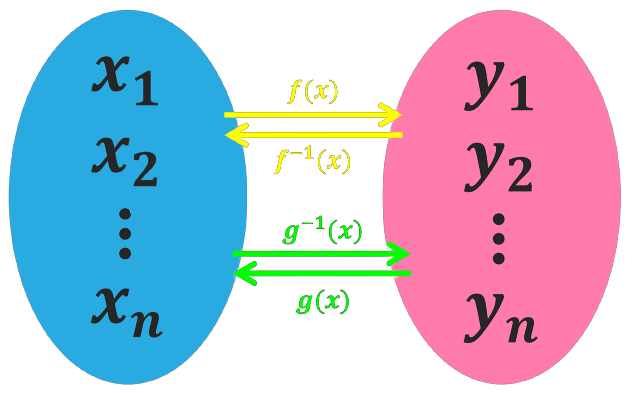### 五、複合函數／合成函數（composite function）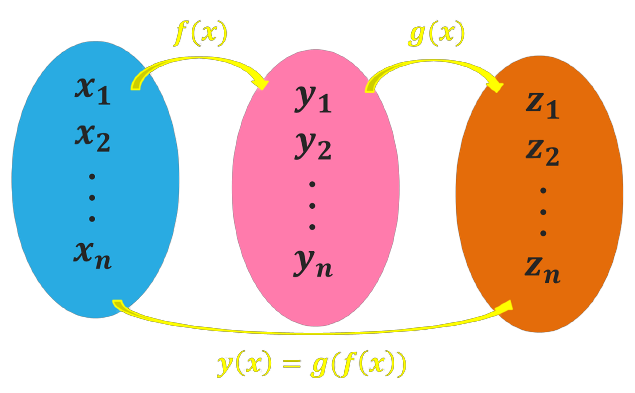## 錯排（derangement）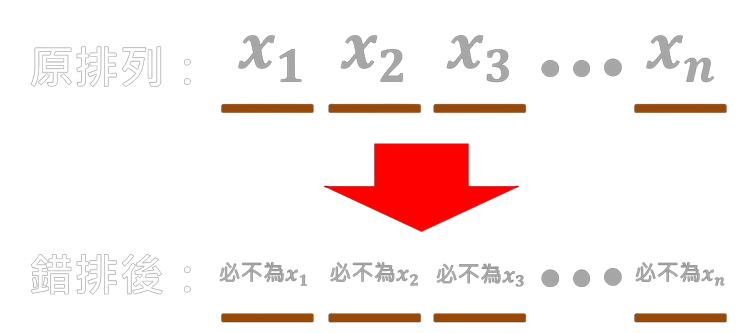#### 錯排數量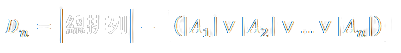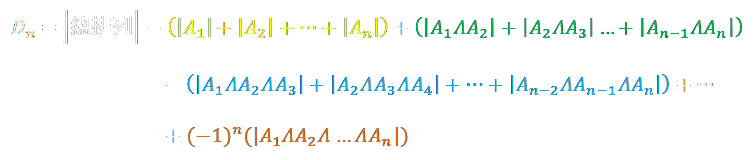1. 白色：錯排前所有排列可能性個數，共有 n! 種。
2. 黃色：有一個數在原位置個數，共有 C(n,1)(n–1)! 種。
3. 綠色：有兩個數在原位置個數，共有 C(n,2)(n–2)! 種。
4. 藍色：有三個數在原位置個數，共有 C(n,3)(n–3)! 種。
5. 橘色：所有數都在原位置個數，共有 C(n,n)(n–n)!=1 種。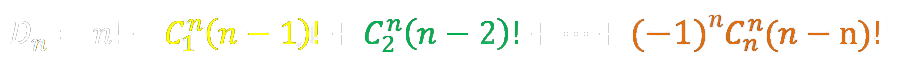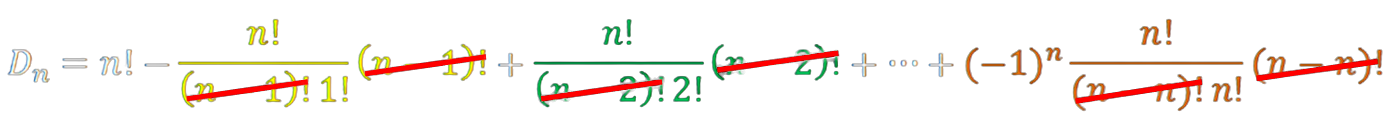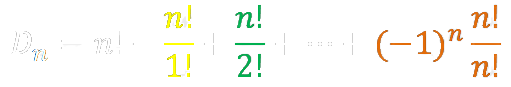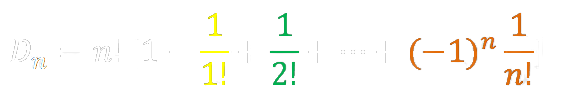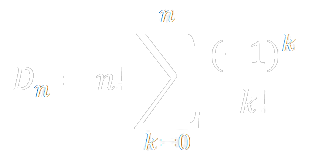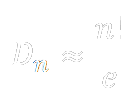## 模除（modulo operation）

1. 3÷3=1…0，因此 3%3=0
2. 2÷3=0...2，因此 2%3=2
3. 1÷3=0…1，因此 1%3=1
4. 0÷3=0…0，因此 0%3=0
5. (-1)÷3=(-1)…2，因此 (-1)%3=2
6. (-2)÷3=(-1)…1，因此 (-2)%3=1
7. (-3)÷3=(-1)…0，因此 (-3)%3=0

### 一、模算數（modular arithmetic）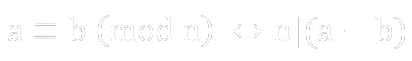1. 5%2=1
2. 3%2=1

1. a–b=5–3=2

#### 模算數原理

1. 餘數為 0 者：{…(-9),(-6),(-3),0,3,6,9…}
2. 餘數為 1 者：{…(-8),(-5),(-2),1,4,7,…}
3. 餘數為 2 者：{...(-7),(-4),(-1),2,5,8…}

## 小結

1. 二元關係（ρ）：同一集合中或相異集合間，兩元素間關係的集合。
2. 齊次關係：描述同一集合內元素自己跟自己、或跟其他元素間的二元關係。
3. 自反性：齊次關係性質之一，描述一集合中某元素自己跟自己有關。
4. 非自反性：齊次關係性質之一，描述一集合中某元素自己跟自己無關。
5. 對稱性：齊次關係性質之一，若一集合中兩元素 (a,b) 有該二元關係，(b,a) 也有該二元關係，則兩元素相等。
6. 反對稱性：齊次關係性質之一，若一集合中兩元素 (a,b)、(b,a) 都有該二元關係，則 a=b。
7. 非對稱性：齊次關係性質之一，若一集合中兩元素 (a,b) 有該二元關係，則 (b,a) 沒有該二元關係。
8. 遞移性：齊次關係性質之一，若一集合中兩元素 (a,b) 及另外兩元素 (b,c) 都有該二元關係，則 (a,c) 也有該二元關係。
9. 弱連通性：齊次關係性質之一，對於一集合中兩元素 a、b，只要兩元素相異，則 (a,b) 或 (b,a) 屬於該二元關係。
10. 強連通性：齊次關係性質之一，對於一集合中兩元素 a、b，(a,b) 或 (b,a) 屬於該二元關係。
11. 關係閉包：對於某一不滿足特定二元關係的集合，經增加最少序對後，形成符合該二元關係的新集合。
12. 偏序關係：同時滿足自反性、反對稱性、遞移性的二元關係。
13. 哈斯圖：用最簡化的方式表示有限偏序關係集合的圖。
14. 實質等價：同時滿足自反性、對稱性、遞移性的二元關係。
15. 函數：兩集合間的二元關係，每個自變數只能對應一個應變數。
16. 自變數=原像：函數輸入值。
17. 應變數=像：函數輸出值。
18. 定義域：自變數的集合。
19. 對應域：應變數存在的集合，包含非應變數與所有應變數。
20. 值域：對應域中，所有應變數的集合。
21. 單射：每個自變數都只對應一個應變數。
22. 單射函數個數：若一函數定義域有 m 個元素、對應域有 n 個元素，m≤n，則有 P(n,m) 個單射函數。
23. 滿射：每個應變數都有自變數與其對應。
24. 滿射函數個數：若一函數定義域有 m 個元素、對應域有 n 個元素，m≥n，則有 nᵐ–[C(n,1)(n–1)ᵐ+C(n,2)(n–2)ᵐ+...+C(n,n)(n–n)ᵐ] 個滿射函數。
25. 雙射：所有自變數跟應變數都是一對一配對。
26. 反函數：函數的逆向運作，如對於函數 f 而言，f⁻¹ 為其反函數；所有反函數皆為雙射函數。
27. 複合函數：若 y(x)=f(g(x))，則 y(x) 為複合函數。
28. 錯排（Dₙ或!n）：排列好的物件重新排列後，每個物件都不在原來的位置上。
29. 錯排數量：可用「排容原理」計算，最終約等於 n!/e。
30. 模除（%）：用於求除法中的餘數。
31. 模算數：若兩整數 a、b 之差可被一正整數 n 整除，若且唯若 a%n=b%n。
#離散數學 #關係 #函數